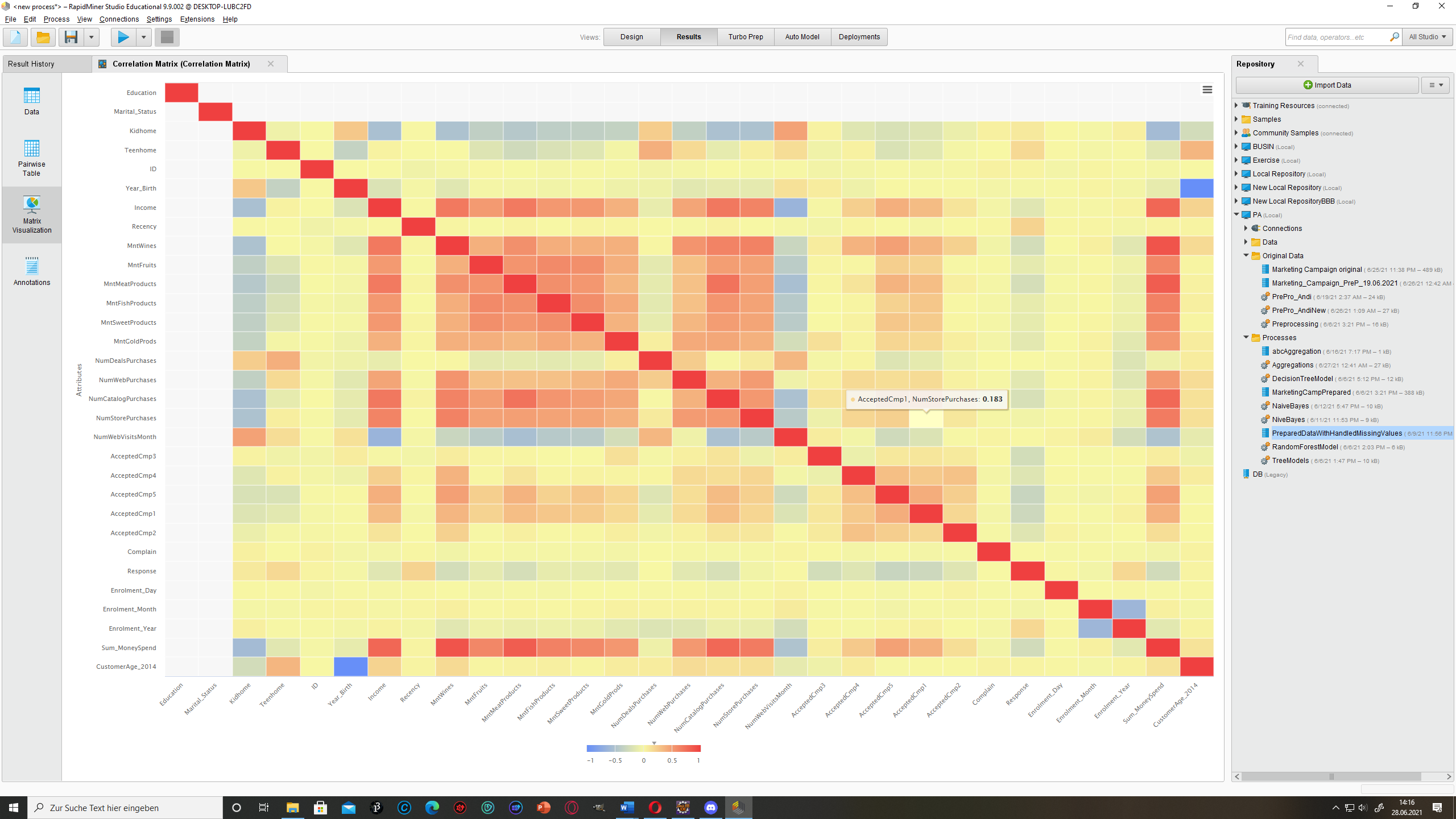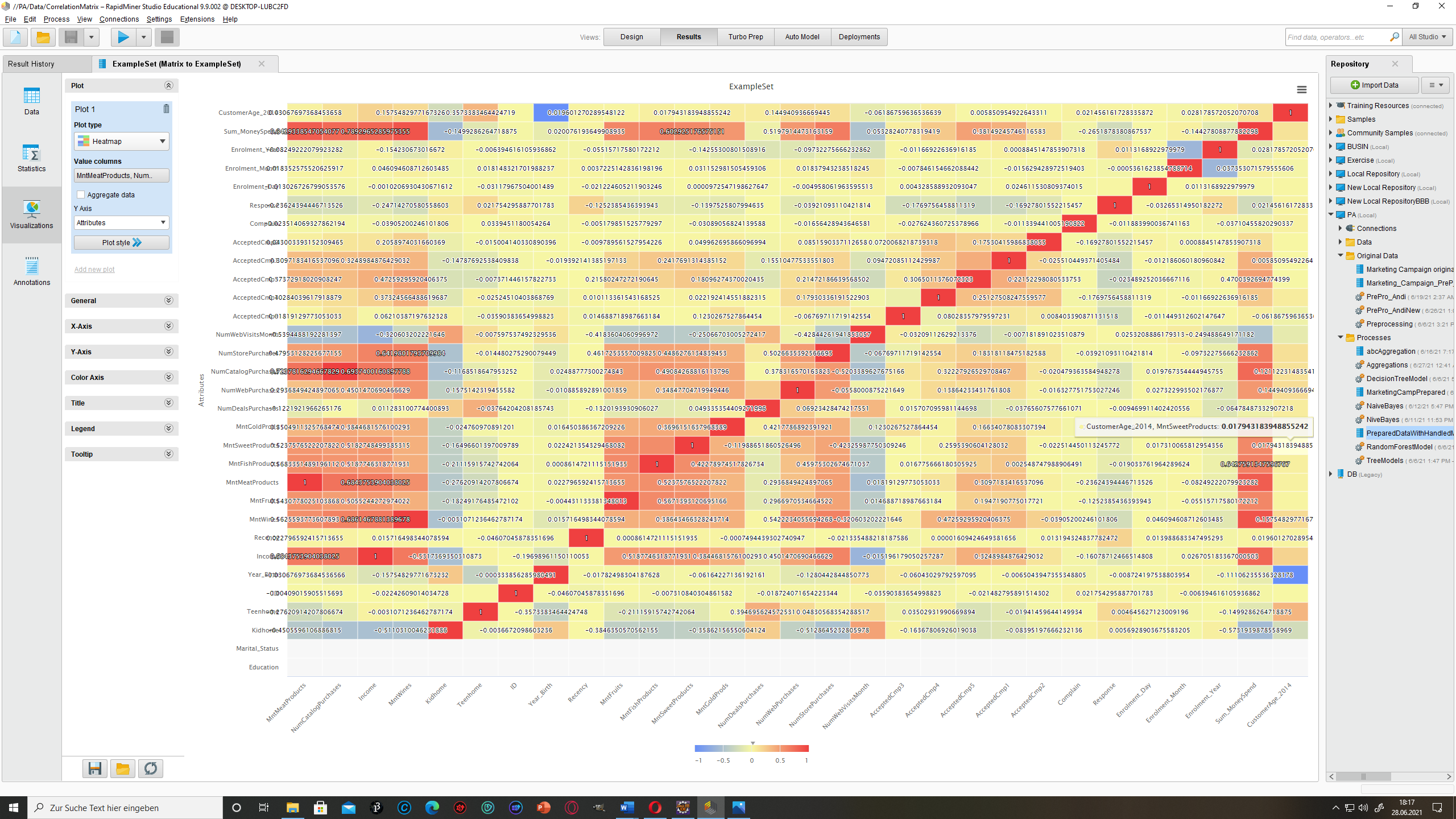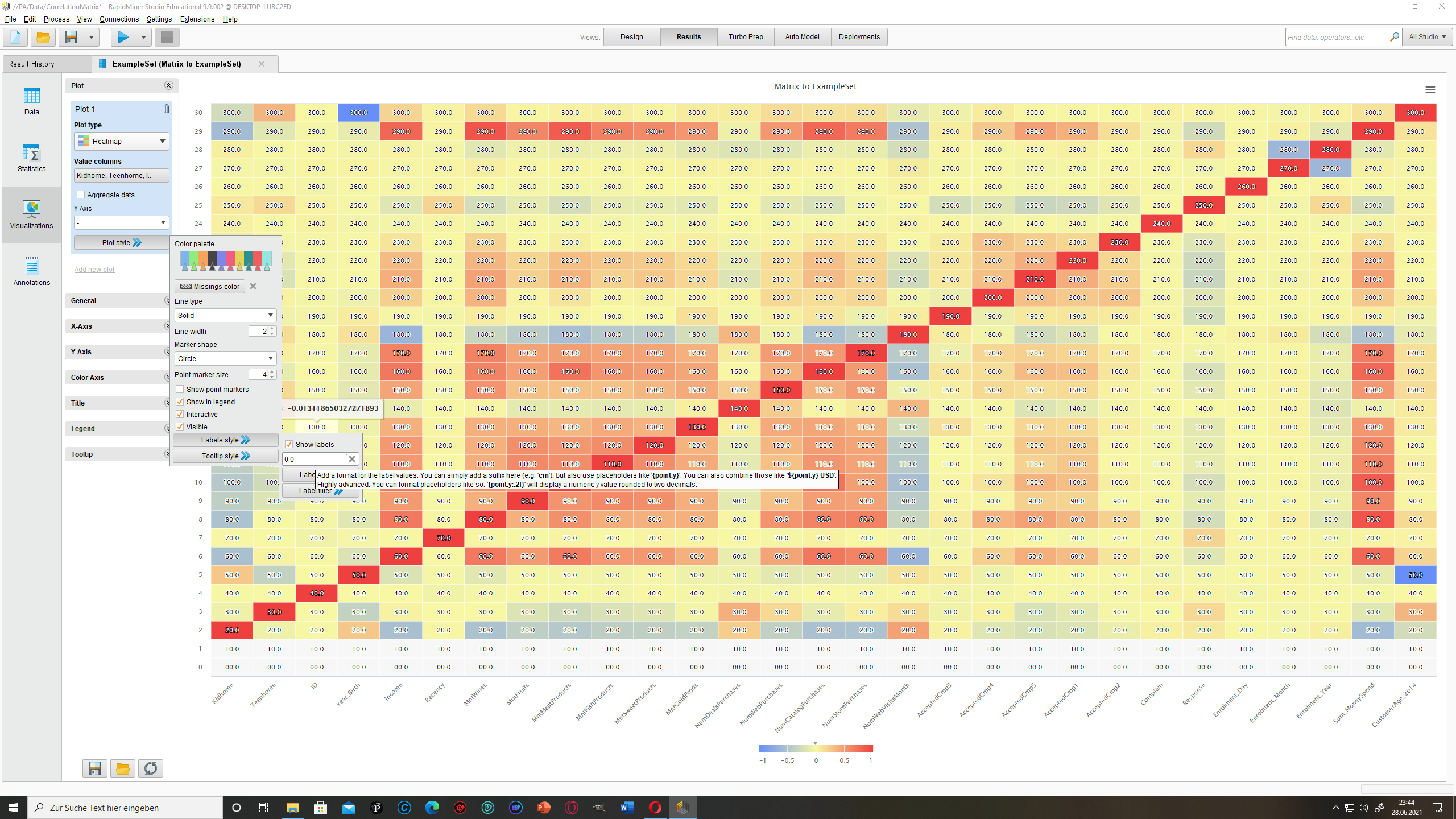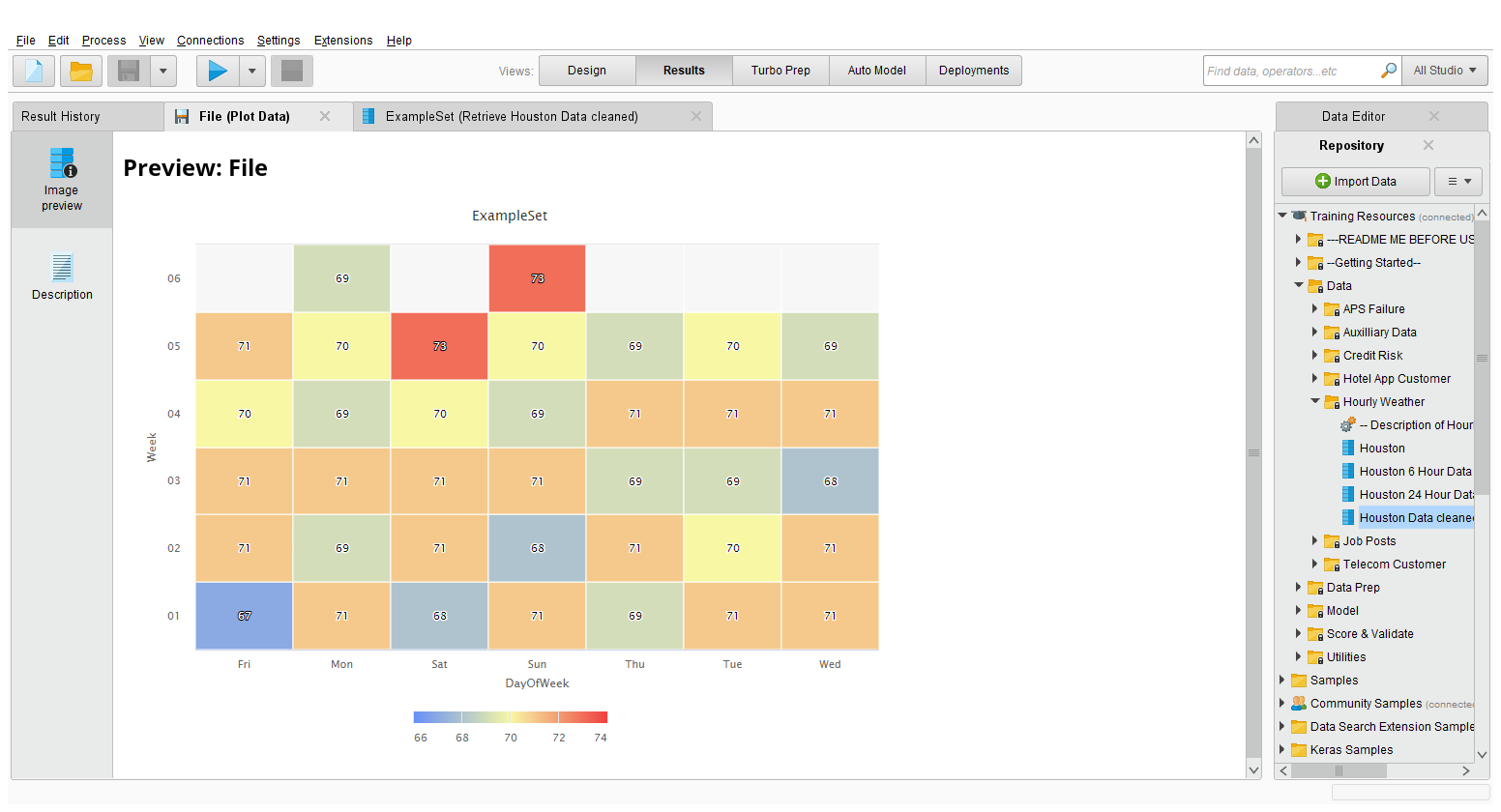# How to show numbers - Visualization

Member Posts: 27Contributor II
Hello,
I'm trying to download the correlation table but it's only showing the numbers if I go over it with my mouse. How do I activate to see all numbers at once?• Administrator, Employee, RapidMiner Certified Analyst, Member Posts: 271Unicorn
Solution Accepted
Hi @Ellie98,

On my response I attached the xml of my base process and a json config file that you will use to set all the parameters for the chart.
The changes are done on the json file, open it with any note pad software. To control the fontSize you simply change the null value to whatever number you want.
The extract of the JSON I showed is already on the file you just need to find it. I think is line 120 of the fie.

• Administrator, Moderator, Employee, RapidMiner Certified Analyst, RapidMiner Certified Expert Posts: 955Unicorn
Hi @Ellie98

you can "select all" by pressing Control+A in the Data or Pairwaise Table and copy to a spreadsheet.

There's also an operator "Matrix to ExampleSet" in the Converters extension which you find in the marketplace. This gives you a table that you can easily write into a database, export into a file or operate on it any way you want in RapidMiner.

Regards,
Balázs
• Member Posts: 27Contributor II
@BalazsBarany That's pretty interesting, thanks!! But there isn't the same plot type like above. Can I download that too somehow?
• Administrator, Moderator, Employee, RapidMiner Certified Analyst, RapidMiner Certified Expert Posts: 955Unicorn
Hi @Ellie98

the picture you sent is a visualization, not a table. It would be cool to have exactly that but with the numbers, but you can't do that in Studio as far as I know.

However, if you export the matrix to a spreadsheet like Libreoffice Calc or Excel, you should be able to apply conditional formatting to get a similar display with the background of the cells coloured according to the values inside.

Regards,
Balázs
• Member Posts: 27Contributor II
It worked. Am I able to cut the huge comma numbers somehow? To only show 3 numbers after a comma @BalazsBarany• Administrator, Moderator, Employee, RapidMiner Certified Analyst, RapidMiner Certified Expert Posts: 955Unicorn
Hi,

you can often enter a format string like 0.00 to style the displayed numbers.

Regards,
Balázs
• Member Posts: 27Contributor II
Hey @BalazsBarany
Am I able to format it with Label style somehow? If I write in 0.0 I get huge numbers thoughBest wishes,
Ellie
• Administrator, Moderator, Employee, Member, University Professor Posts: 1,985RM Engineering
edited June 2021
Hi,

You can style the numbers by entering the following into the label format value field:{point.y:.0f}
If you replace the 0 with a 1, you would get a fractional digit, and so on.

Regards,
Marco
• Administrator, Employee, RapidMiner Certified Analyst, Member Posts: 271Unicorn
edited June 2021
Hi @Ellie98,

Please see if the example I'm sharing is what you expect as an output.

You'll need to install the Animated Plots extension and use the config file I'm sharing.

Whit it you have fool control and automation on the graphs and you can tweak your config in an "easy and replicable" way.

The only thing I changed from the default RM json was
"dataLabelConfiguration" : {
"enabled" : true,
"format" : null,
"style" : {
"color" : null,
"fontSize" : null,
"bold" : false,
"italic" : false
},```<?xml version="1.0" encoding="UTF-8"?><process version="9.9.002">
<context>
<input/>
<output/>
<macros/>
</context>
<operator activated="true" class="process" compatibility="9.9.002" expanded="true" name="Process">
<parameter key="logverbosity" value="init"/>
<parameter key="random_seed" value="-1"/>
<parameter key="send_mail" value="never"/>
<parameter key="process_duration_for_mail" value="30"/>
<parameter key="encoding" value="SYSTEM"/>
<process expanded="true">
<operator activated="true" class="retrieve" compatibility="9.9.002" expanded="true" height="68" name="Retrieve Houston Data cleaned" width="90" x="112" y="34">
<parameter key="repository_entry" value="//Training Resources/Data/Hourly Weather/Houston Data cleaned"/>
</operator>
<operator activated="true" class="generate_copy" compatibility="9.9.002" expanded="true" height="82" name="week" width="90" x="246" y="34">
<parameter key="attribute_name" value="datetime"/>
<parameter key="new_name" value="Week"/>
</operator>
<operator activated="true" class="generate_copy" compatibility="9.9.002" expanded="true" height="82" name="month" width="90" x="380" y="34">
<parameter key="attribute_name" value="datetime"/>
<parameter key="new_name" value="DayOfWeek"/>
</operator>
<operator activated="true" class="date_to_nominal" compatibility="9.9.002" expanded="true" height="82" name="Date to Nominal" width="90" x="45" y="136">
<parameter key="attribute_name" value="Week"/>
<parameter key="date_format" value="WW"/>
<parameter key="time_zone" value="SYSTEM"/>
<parameter key="locale" value="English (United States)"/>
<parameter key="keep_old_attribute" value="false"/>
</operator>
<operator activated="true" class="date_to_nominal" compatibility="9.9.002" expanded="true" height="82" name="Date to Nominal (2)" width="90" x="179" y="187">
<parameter key="attribute_name" value="DayOfWeek"/>
<parameter key="date_format" value="E"/>
<parameter key="time_zone" value="SYSTEM"/>
<parameter key="locale" value="English (United States)"/>
<parameter key="keep_old_attribute" value="false"/>
</operator>
<operator activated="true" class="aggregate" compatibility="9.9.002" expanded="true" height="82" name="Aggregate" width="90" x="313" y="187">
<parameter key="use_default_aggregation" value="false"/>
<parameter key="attribute_filter_type" value="all"/>
<parameter key="attribute" value=""/>
<parameter key="attributes" value=""/>
<parameter key="use_except_expression" value="false"/>
<parameter key="value_type" value="attribute_value"/>
<parameter key="use_value_type_exception" value="false"/>
<parameter key="except_value_type" value="time"/>
<parameter key="block_type" value="attribute_block"/>
<parameter key="use_block_type_exception" value="false"/>
<parameter key="except_block_type" value="value_matrix_row_start"/>
<parameter key="invert_selection" value="false"/>
<parameter key="include_special_attributes" value="false"/>
<parameter key="default_aggregation_function" value="average"/>
<list key="aggregation_attributes">
<parameter key="Temperature / Fahrenheit" value="average"/>
</list>
<parameter key="group_by_attributes" value="DayOfWeek|Week"/>
<parameter key="count_all_combinations" value="false"/>
<parameter key="only_distinct" value="false"/>
<parameter key="ignore_missings" value="true"/>
</operator>
<operator activated="true" class="generate_attributes" compatibility="9.9.002" expanded="true" height="82" name="Generate Attributes" width="90" x="447" y="187">
<list key="function_descriptions">
<parameter key="average(Temperature / Fahrenheit)" value="round([average(Temperature / Fahrenheit)],0)"/>
</list>
<parameter key="keep_all" value="true"/>
</operator>
<operator activated="true" class="open_file" compatibility="9.9.002" expanded="true" height="68" name="Open File" width="90" x="313" y="340">
<parameter key="resource_type" value="file"/>
</operator>
<operator activated="true" class="animated_plots:plotHtml5" compatibility="9.9.000" expanded="true" height="82" name="Plot Data" width="90" x="581" y="238">
<parameter key="width" value="800"/>
<parameter key="height" value="600"/>
</operator>
<connect from_op="Retrieve Houston Data cleaned" from_port="output" to_op="week" to_port="example set input"/>
<connect from_op="week" from_port="example set output" to_op="month" to_port="example set input"/>
<connect from_op="month" from_port="example set output" to_op="Date to Nominal" to_port="example set input"/>
<connect from_op="Date to Nominal" from_port="example set output" to_op="Date to Nominal (2)" to_port="example set input"/>
<connect from_op="Date to Nominal (2)" from_port="example set output" to_op="Aggregate" to_port="example set input"/>
<connect from_op="Aggregate" from_port="example set output" to_op="Generate Attributes" to_port="example set input"/>
<connect from_op="Generate Attributes" from_port="example set output" to_op="Plot Data" to_port="example set"/>
<connect from_op="Open File" from_port="file" to_op="Plot Data" to_port="chart configuration"/>
<connect from_op="Plot Data" from_port="img" to_port="result 1"/>
<portSpacing port="source_input 1" spacing="0"/>
<portSpacing port="sink_result 1" spacing="0"/>
<portSpacing port="sink_result 2" spacing="0"/>
</process>
</operator>
</process>```

• Member Posts: 27Contributor II